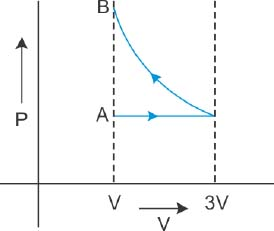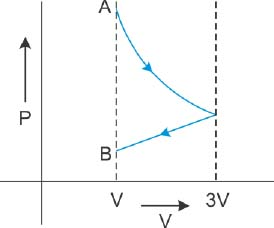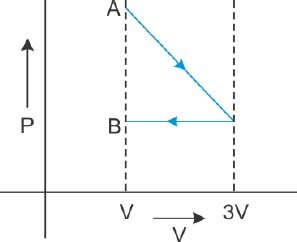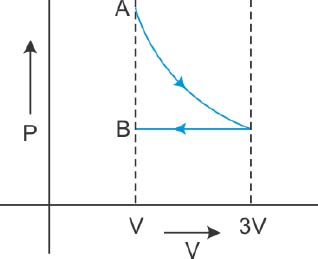# One mole of an ideal gas goes from an initial state A to final state B via two processes: It first undergoes isothermal expansion from volume V to 3V and then its volume is reduced from 3V to V at constant pressure. The correct P-V diagram representing the two processes is : Option 1)Option 2)Option 3)Option 4)As we learnt in

Isobaric process -

A Thermodynamic process in which pressure remain constant.

- wherein

First process is V 3V (Isothermal)

Second Process is 3V  V(Isobaric)

Correct option is 4.

Option 1)This is incorrect option

Option 2)This is incorrect option

Option 3)This is incorrect option

Option 4)This is correct option

Exams
Articles
Questions